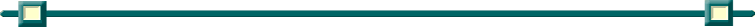Finding Maxima and Minima
for simple functions

METHOD 1: Investigate relative minima/maxima for y = (x - 2)2 + 1.

Step 1: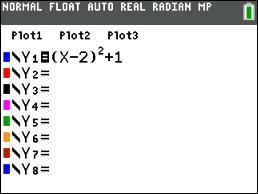Enter the equation into Y=

Step 2:Hit GRAPH.
It may be necessary to adjust the WINDOW so you can see the "hills" and "valleys".

Step 3: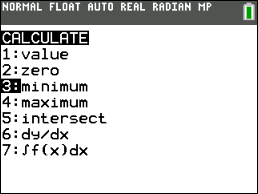This graph has a "valley", so look for the minimum.  Under the CALC (2nd TRACE) menu, choose #3 minimum.
Hit ENTER.

Step 4:When asked for Left Bound, move the cursor (use arrow keys) to the left of the observed minimum location.  Hit ENTER.  You will see a mark indicating that you have "locked" this position.

Step 5: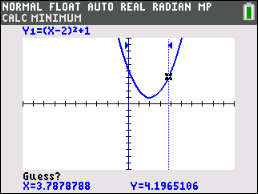When asked for Right Bound, move the cursor (use arrow keys) to the right of the observed minimum location.  Hit ENTER.  You will see a mark indicating that you have "locked" this position.

Step 6: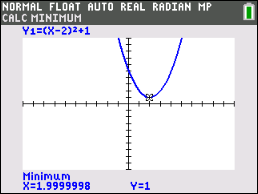When the last screen asked for Guess, simply hit ENTER.

Step 7:The coordinates of the miminum value (within your marked boundaries) is:
Min (2, 1)Note:   If you are investigating more than one location on a graph, you must start this process from the beginning for each point being investigated.  (Return to Step 3.)

This same process is used to examine relative maxima.
In step 3, choose #4: maximum.

METHOD 2: Investigate relative minima/maxima for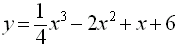.

Step 1: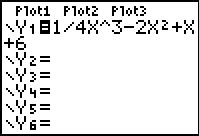Enter the equation into Y=

Step 2:Hit GRAPH.  Be sure the graph is viewable in the graphing window.  Adjust the WINDOW if needed.

Step 3: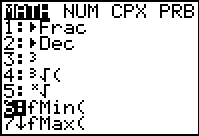From the HOME screen, hit the MATH key.  Choose either #6 fMin or #7 fMax.
Hit ENTER

Step 4: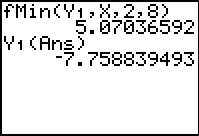The parameters for fMin and fMax the same:
fMin(expression, variable, left bound, right bound)
Be careful:  The answer from fMin is the X-coordinate where the minimum occurs.  It is not the actual y-value minimum.   You must then calculate the y-value.

Step 5:Again, remember that the answer from fMax is the X-coordinate where the maximum occurs.  You must then calculate the y-value.

 HINT: To get Y1 (Ans)    Y1:   VARS → Y-VARS              #1Function    Ans:   2nd (-) key